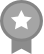`formally known as rainbowsidemen!!s i x t e e n | s h e  /  h e r ___ r u b yd r e a m   s m p/ s i d e m e n / f a l s e t t o s / h a r r y  p o t t e r [i just write books and fangirl]@raintopia_ on instagram and twitterc u r r e n t.• r o a d  t r i p [ d n f ]• n o  c h o i c e [ d r a c o ]c o m p l e t e d.• p r  s t u n t [ s d m n ]• m i s s i n g [ s d m n ]• a f t e r l i f e [ s d m n ]• w r i t t e n [ s d m n ]o n  h o l d.• t h e  w a y  i t  e n d e d [ s d m n ]• s i d e m e n  i m a g i n e s [ s d m n ] [ messing around on here since 5 / 9 / 1 8 ]`
• snowchester w/ michael
• JoinedSeptember 5, 2018

Following

Last Messageraintopia May 10, 2021 03:46PM
`@harrypctter how do i face reveal to you `

Stories by •#12 in road See all rankings#166 in ao3 See all rankings#618 in high See all rankings